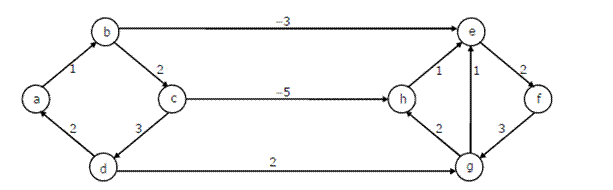# Algorithms | Graph Shortest Paths | Question 3

Dijkstra’s single source shortest path algorithm when run from vertex a in the below graph, computes the correct shortest path distance to(A) only vertex a
(B) only vertices a, e, f, g, h
(C) only vertices a, b, c, d
(D) all the vertices

Explanation: Dijkstra’s single source shortest path is not guaranteed to work for graphs with negative weight edges, but it works for the given graph.
Let us see…

Let us run the 1st pass
b 1
b is minimum, so shortest distance to b is 1.

After 1st pass, distances are
c 3, e -2.
e is minimum, so shortest distance to e is -2

After 2nd pass, distances are
c 3, f 0.
f is minimum, so shortest distance to f is 0

After 3rd pass, distances are
c 3, g 3.
Both are same, let us take g. so shortest distance to g is 3.

After 4th pass, distances are
c 3, h 5
c is minimum, so shortest distance to c is 3

After 5th pass, distances are
h -2
h is minimum, so shortest distance to h is -2

Quiz of this Question

My Personal Notes arrow_drop_up

Article Tags :

Be the First to upvote.

Please write to us at contribute@geeksforgeeks.org to report any issue with the above content.